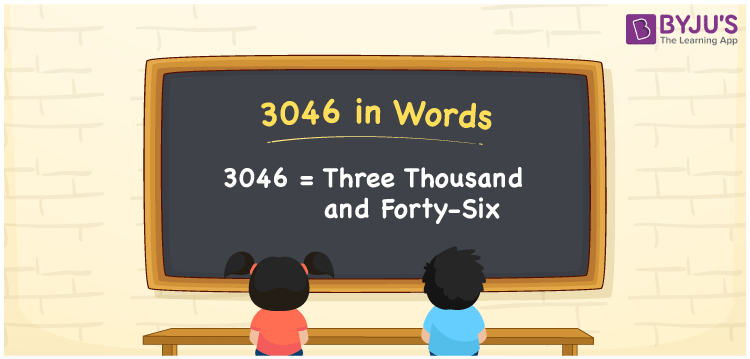# 3046 in Words

The number 3046 in words is written as Three thousand forty-six. In both the International and Indian System of Numerals, 3046 is written as 3,046 and read as Three thousand forty-six. We may use 3046 as “that gadget costs 3046 rupees” in this context, 3046 represents an amount; that is why 3046 is a Cardinal Number since it describes some quantity.

 3046 in Words Three thousand forty-six Three thousand forty-six in Numbers 3046

## 3046 in English Words

In English words, 3046 is written as “Three thousand forty-six”.## How to Write 3046 in Words?

To write 3046 in words, we shall use the place value. In the place value chart, write 3 in thousands, 0 in hundreds, 4 in tens and 6 in ones. Let us make a place value chart to write the number 3046 in words.

 Thousands Hundreds Tens Ones 3 0 4 6

Thus, we can the expanded form as

3 × Thousand + 0 × Hundred + 4 × Ten + 6 × One

= 3 × 1000 + 0 × 100 + 4 × 10 + 6 × 1

= 3000 + 0 + 40 + 6

= 3046

= Three thousand forty-six.

3046 is a Natural Number, the successor of 3045 and the predecessor of 3047

3046 in words – Three thousand forty-six

• Is 3046 an odd number? – No
• Is 3046 an even number? – Yes
• Is 3046 a perfect square number? – No
• Is 3046 a perfect cube number? – No
• Is 3046 a prime number? – No
• Is 3046 a composite number? – Yes

## Frequently Asked Questions on 3046 in Words

Q1

### How to write 3046 in words?

3046 in words is written as Three thousand forty-six.
Q2

### How to write 3046 in the International and Indian System of Numerals?

In both, the system of numerals, 3046 in words, is written as Three thousand forty-six.
Q3

### How to write 3046 in a place value chart?

In the place value chart, write 3 in thousands, 0 in hundreds, 4 in tens and 6 in ones, respectively.